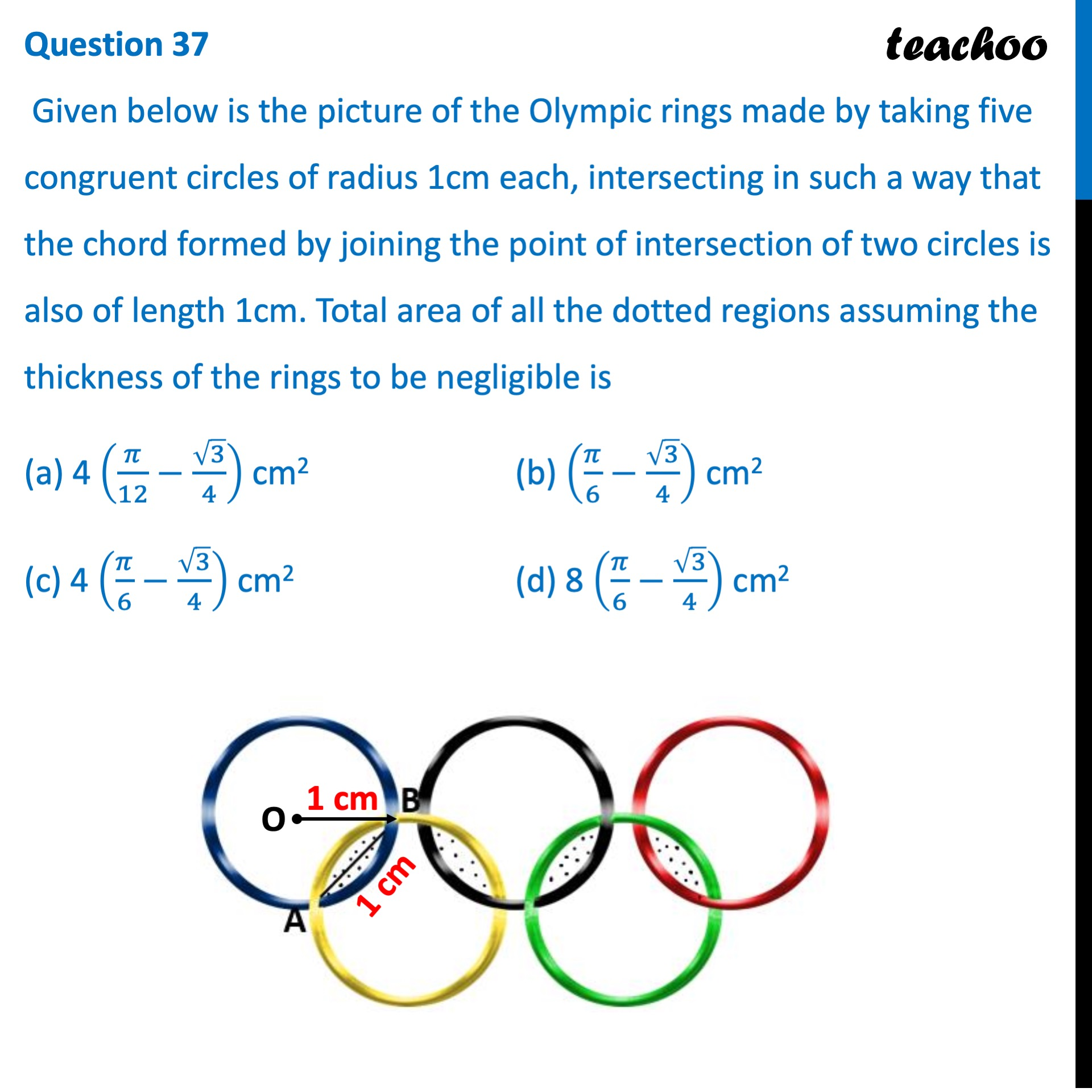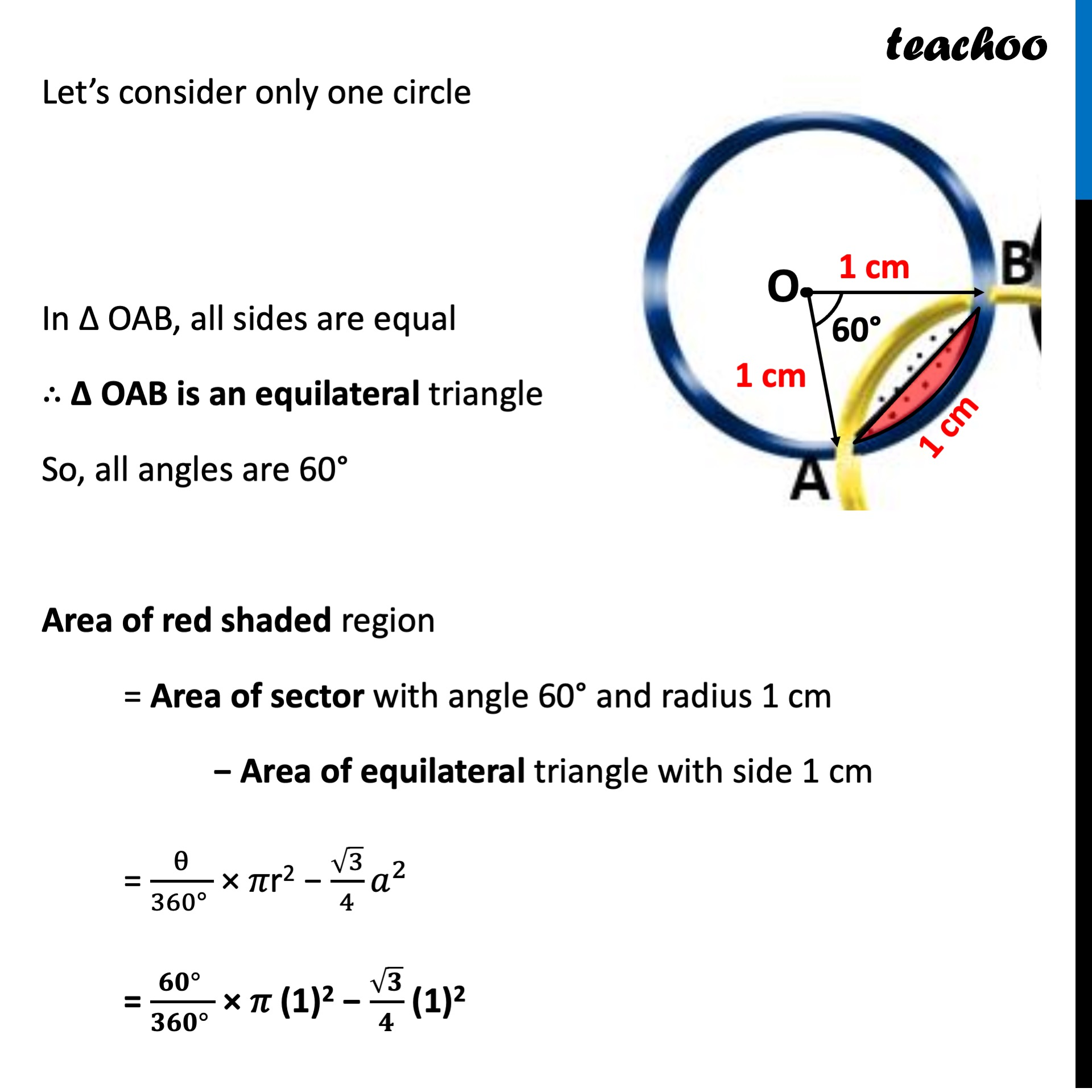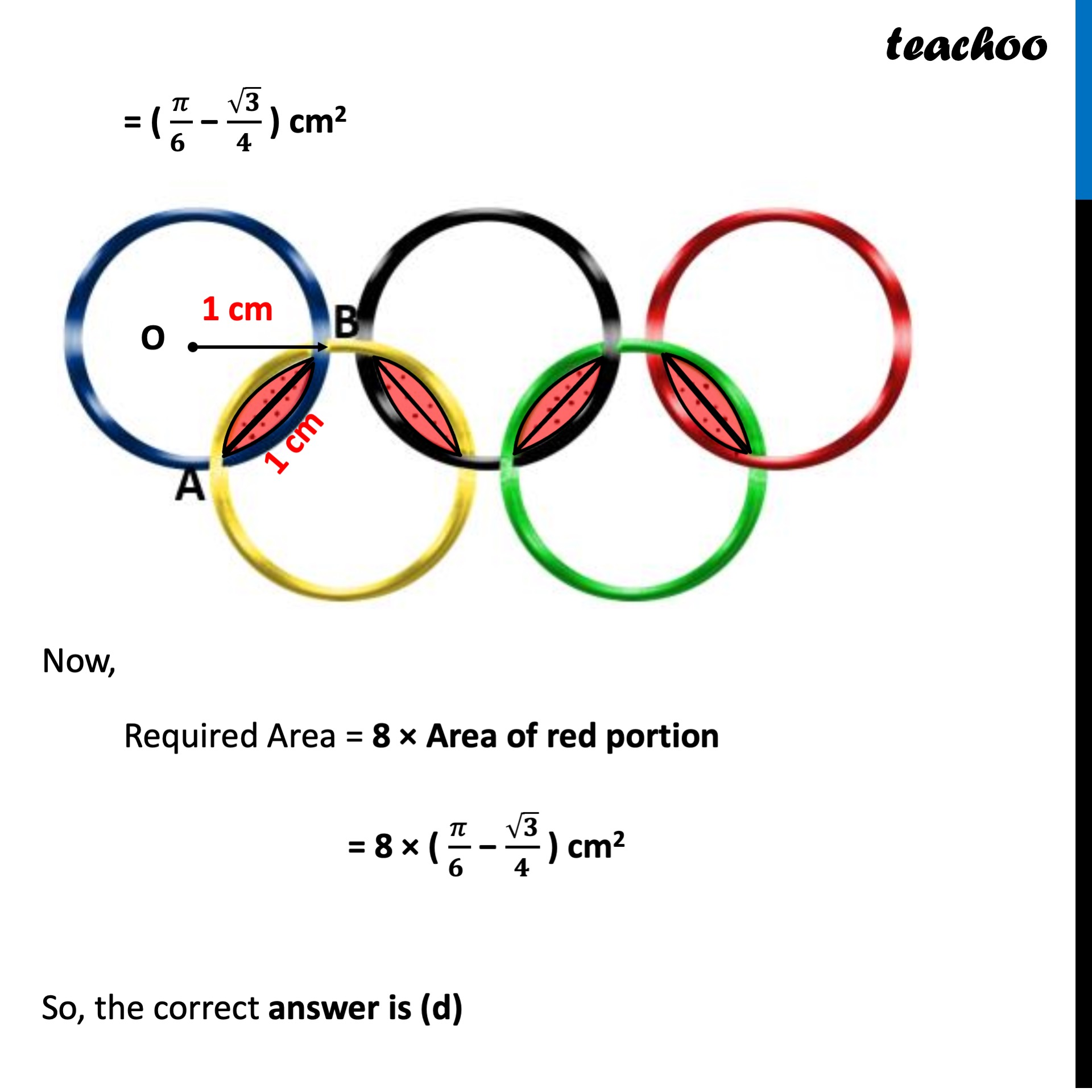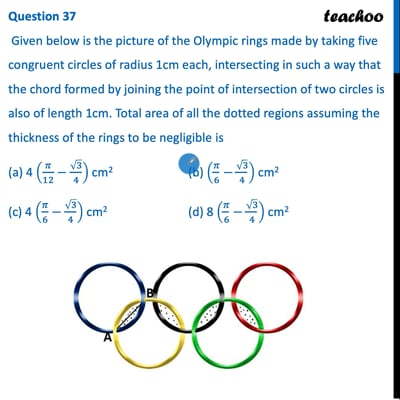CBSE Class 10 Sample Paper for 2022 Boards - Maths Standard [MCQ]

Class 10
Solutions of Sample Papers for Class 10 Boards

## (a) 4 (π/12-√3/4)  cm 2  (b) (π/6-√3/4)  cm 2 (c) 4 (π/6-√3/4)  cm 2  (d) 8 (π/6-√3/4)  cm 2This video is only available for Teachoo black users

### Transcript

Question 37 Given below is the picture of the Olympic rings made by taking five congruent circles of radius 1cm each, intersecting in such a way that the chord formed by joining the point of intersection of two circles is also of length 1cm. Total area of all the dotted regions assuming the thickness of the rings to be negligible is (a) 4 (𝜋/12−√3/4) cm2 (b) (𝜋/6−√3/4) cm2 (c) 4 (𝜋/6−√3/4) cm2 (d) 8 (𝜋/6−√3/4) cm2 Let’s consider only one circle In Δ OAB, all sides are equal ∴ Δ OAB is an equilateral triangle So, all angles are 60° Area of red shaded region = Area of sector with angle 60° and radius 1 cm − Area of equilateral triangle with side 1 cm = θ/(360° ) × 𝜋r2 − √3/4 𝑎^2 = (𝟔𝟎° )/(𝟑𝟔𝟎° ) × 𝜋 (1)2 − √𝟑/𝟒 (1)2 = ( 𝜋/(𝟔 ) − √𝟑/𝟒 ) cm2 Now, Required Area = 8 × Area of red portion = 8 × ( 𝜋/(𝟔 ) − √𝟑/𝟒 ) cm2 So, the correct answer is (d)